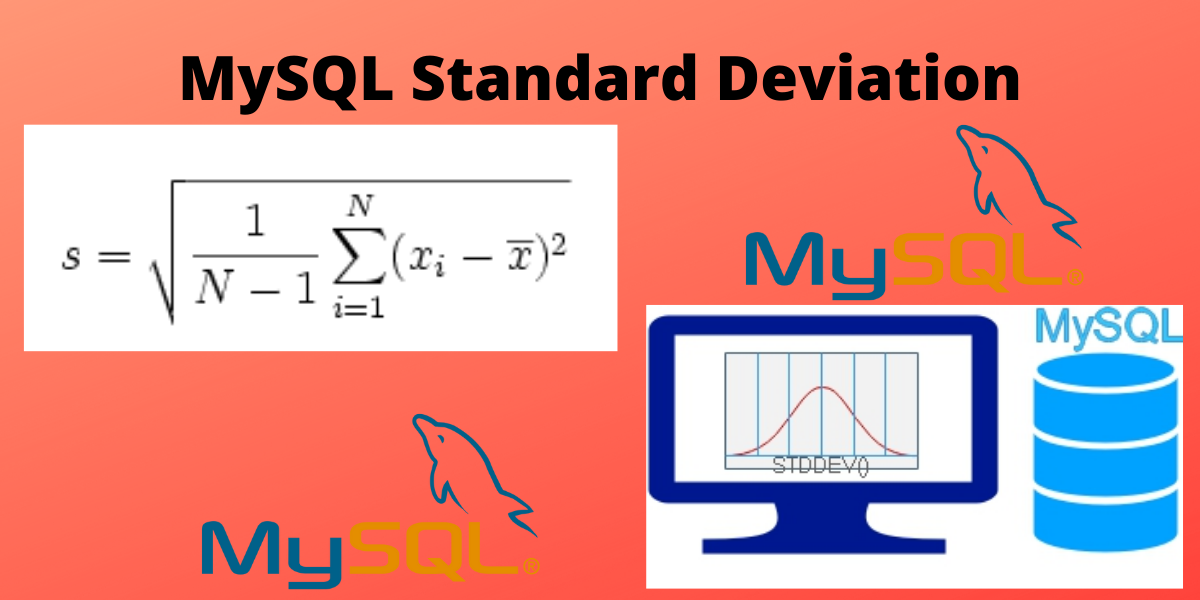# MySQL Standard Deviation – A Beginner’s GuideIn this tutorial, we will study standard deviation and functions to calculate standard deviation in MySQL.

## Introduction to Standard Deviation

The standard deviation calculates how much data is spread over the mean value. It basically tells how much variation is there in the dataset. When the standard deviation is low then it means that the values of the data set are close to the mean and if the standard deviation is high then it means that the values of the data set are spread over a large range of values. From this, we can infer that a low standard deviation implies higher precision and accuracy.

One of the key applications of the standard deviation is in statistics. It is used to measure how far the data points are from the mean value. This information can be useful when analyzing statistical or financial data. For example, if the standard deviation of sales performance is low, it means that the sales figures are close to the mean and there is less variation in them. This would indicate that the sales performance is likely to be more consistent and predictable than if the standard deviation were high.

## How to calculate standard deviation

• First of all, calculate the average of all the values in the data set to get the average or mean.
• Calculate the difference of the value from the mean found for each value and square the result of each value.
• In the above step, variance is found. Now take the square root of the variance to get the standard deviation of all the values.

The formula for standard deviation is-

## MySQL Standard Deviation – Methods in MySQL

There are two types of the standard deviation, the population standard deviation and the sample standard deviation. The population standard deviation is used when the entire population can be measured. The sample standard deviation is used when only a sample from the population can be measured.

To calculate population standard deviation-

• STD(expression) – Returns the population standard deviation of the dataset and returns NULL if there are no matching values.
• STDDEV(expression) – Same as the above but works only with Oracle Database.
• STDEV_POP(expression) – This is same as STD function.

To calculate the sample standard deviation, use the function – STDDEV_SAMP (expression).

There are some functions in MySQL, which are helpful to calculate the variance for population and sample variance.

• VAR_POP(expression) – calculates the population standard variance.
• VARIANCE(expression)
• VAR_SAMP(expression) – calculates the sample standard variance.

## Example of MySQL Standard Deviation

Here we will use the classicmodels database to calculate the standard deviation. In the database, we will use the orders table. We are calculating population standard deviation.

First, we will count the number of orders using the customerNumber field. The code for the following is-

```.wp-block-code {
border: 0;
}

.wp-block-code > span {
display: block;
overflow: auto;
}

.shcb-language {
border: 0;
clip: rect(1px, 1px, 1px, 1px);
-webkit-clip-path: inset(50%);
clip-path: inset(50%);
height: 1px;
margin: -1px;
overflow: hidden;
position: absolute;
width: 1px;
word-wrap: normal;
word-break: normal;
}

.hljs {
box-sizing: border-box;
}

.hljs.shcb-code-table {
display: table;
width: 100%;
}

.hljs.shcb-code-table > .shcb-loc {
color: inherit;
display: table-row;
width: 100%;
}

.hljs.shcb-code-table .shcb-loc > span {
display: table-cell;
}

.wp-block-code code.hljs:not(.shcb-wrap-lines) {
white-space: pre;
}

.wp-block-code code.hljs.shcb-wrap-lines {
white-space: pre-wrap;
}

.hljs.shcb-line-numbers {
border-spacing: 0;
counter-reset: line;
}

.hljs.shcb-line-numbers > .shcb-loc {
counter-increment: line;
}

.hljs.shcb-line-numbers .shcb-loc > span {
}

.hljs.shcb-line-numbers .shcb-loc::before {
border-right: 1px solid #ddd;
content: counter(line);
display: table-cell;
text-align: right;
-webkit-user-select: none;
-moz-user-select: none;
-ms-user-select: none;
user-select: none;
white-space: nowrap;
width: 1%;
}```Select customerNumber,
count(*) totalOrders
From orders
Where status = 'Shipped'
Group By customerNumber;```Code language: SQL (Structured Query Language) (sql)```

Output-

Now we will calculate the population standard deviation of the number of orders of the customers-

``````Select Format(STD(totalOrders), 2)
From (Select customerNumber, count(*) totalOrders
From orders
Group By customerNumber) a;```Code language: SQL (Structured Query Language) (sql)```

Output-

Now we will calculate Sample standard deviation. The code for the following is-

``````Select customerNumber, count(*) totalOrders
From orders
Where status = 'Shipped'
Group By customerNumber;

Select FORMAT(STDDEV_SAMP(totalOrders), 2)
From (Select customerNumber, count(*) totalOrders
From orders
Where status = 'Shipped'
Group By customerNumber) b;```Code language: SQL (Structured Query Language) (sql)```

Output-